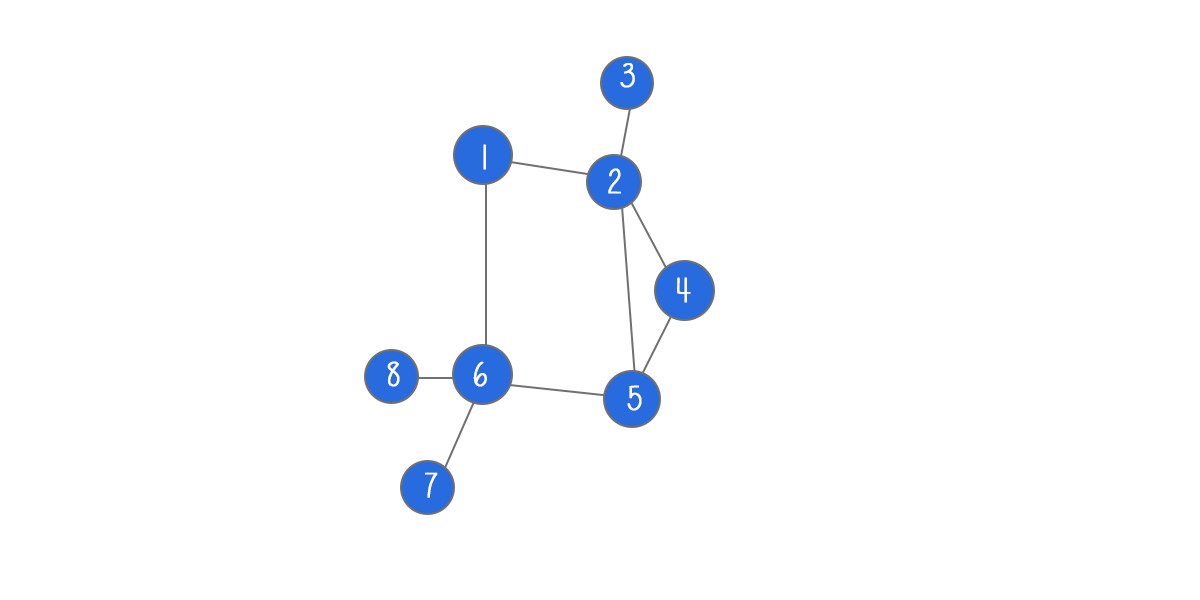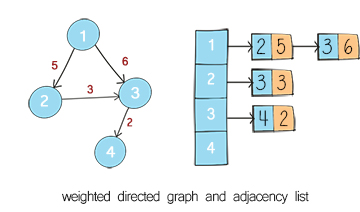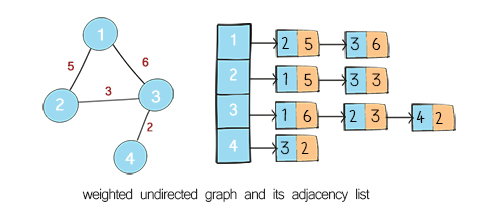A graph is a data structure that consists of a set of  nodes connected by edges. Graphs are used to simulate many real-world problems, such as paths in cities, circuit networks, and social networks. This is graph implementation part 2 – weighted graph.A node in the graph is also called vertex.  A line between two nodes is edge. Two nodes are adjacent (or neighbors) if they are connected to each other through an edge. Path represents a sequence of edges between the two nodes.

In a directed graph, all of the edges represent a one-way relationship. In an undirected graph, all edges are bi-directional.

If the edges in the graph have weights, the graph is said to be a weighted graph. If the edges do not have weights, the graph is said to be unweighted.

The weight of the edges might represent the distances between two cities, or the cost of flights etc. When we include weight as a feature of graph’s edges, some interesting questions arise. The problems such as finding shortest path or longest path are applied to weighted graphs.

Weighted graph can be directed or undirected. For example, the minimum spanning tree is undirected graph. The famous Dijkstra’s algorithm to find shortest path is for directed graphs.

Graph can be presented as adjacent list or adjacent matrix. An adjacent list is an array of edges or nodes. Adjacency list is used for representation of the sparse graphs and used more often. An adjacent matrix is a square matrix with dimensions equivalent to the number of nodes in the graph. Adjacent matrix is preferred when the graph is dense.Part 1 – unweighted, directed/ undirected graph, represented as adjacent list.
Part 2 – weighted, directed/undirected graph, represented as adjacent list.
Part 3 – weighted/unweighted, directed/undirected graph, represented as adjacent matrix

### Define classes

First we define an Edge class. It has two fields: connectedVertex and weight. The connectedVertex is the node at the end of the edge. weight is the value associated with the edge.

The GraphWeighted class has two fields: adj and directed. adj is a HashMap in which the key is the node at the start of the edge, the value is all its neighbors. The value is represented as linked list of the edges. directed is a boolean variable to specify whether the graph is directed or undirected. By default, it is undirected.

The nodes can be any data type, for example primitive data type, such as integer or string. Or it can be an object, such as graphNode.

## Python

To add a node to the graph is to add a key in the hashmap. To add an edge is to add an item in this key’s value. The following method addEdge includes both adding a node and adding an edge. For a directed graph, we add edge from a to b. For undirected graph, we also add edge from b to a. Note the weight is one of the input and used to create edge object.

## Python

### Remove

Remove operation includes remove edge and remove node. Before we continue, let’s create a utility method to find the edge between two nodes. Use one node as key to find its neighbors. Then loop through the neighbors to find the other node. Return the edge object with the weight. This method will be used in following operations.

To remove edge, we use the node as key to find its neighbors in the hashmap. Then remove the other node from its neighbors. For a directed graph, we just need to remove edge from a to b. For an undirected graph, we also need to remove the edge from b to a.

Remove node has more work to do than remove edge. We have to remove all connected edge before remove the node itself. For an undirected graph, first we get all neighbors of the node. Then for each of its neighbors, remove itself from the value list. For a directed graph, we search all keys in the hashmap for their values, and check whether this node exists in their neighbors. If it does, remove it. The last step is to remove the node as the key in the hashmap. Then this node is no longer in the hashmap’s key set.

## Python

### Search

Search can be search node, edge or path. We can check whether there is a node existing in the graph. This can be done by simply checking the hashmap contains the key. We can also check whether there is a direct connection between two nodes (aka whether there is an edge). This can be done by checking whether the other node is in one node’s neighbors.

## Python

More useful operation is to search path. A path is a sequence of edges. There can be more than one path between two nodes. There are two common approaches: depth first search (DFS) and breadth first search (BFS). In this section, we use DFS and BFS to find out whether there is path from one node to another. In “Print and traversal” section, we use them to find all reachable nodes from the source node in graph.

Depth First Search starts from the source node, and explores the adjacent nodes as far as possible before call back. DFS is usually implemented with recursion or stack. It is used to solve find path or detect cycle problems.

## Python

Breath First Search starts from the source node, and explores all its adjacent nodes before going to the next level adjacent nodes. BFS is usually implemented with Queue. It is often used to solve shortest path problems.

## Python

### Print and traversal

Print is to visit all nodes in the graph and print the information stored. Three ways are introduced here.

(1) Print all nodes and their neighbors in the hashmap. This can be done by looping through the key set of the hashmap. This method is used for debugging purpose.

## Python

(2) DFS traversal: Use depth first search to visit nodes in the graph and print the node’s information. This is similar to DFS traversal in binary tree. Starting from the source node, we call recursive method to visit its neighbor’s neighbor until call back. Please node the source might be any node in the graph. Some nodes might not be reached in a directed graph. (In binary tree, we always start from the root and all nodes should be visited.)

## Python

(3) BFS traversal: Use breadth first search to visit all nodes in the graph and print the node’s information. This is similar to BFS traversal in binary tree. Starting from the source, visit all its neighbors first before visiting neighbor’s neighbor. Please node the source might be any node in the graph. Some nodes might not be reached in a directed graph. (In binary tree, we always start from the root and all nodes should be visited.)